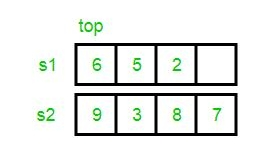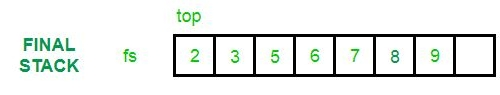Related Articles

# Merging and Sorting Two Unsorted Stacks

• Difficulty Level : Medium
• Last Updated : 31 Aug, 2021

Given 2 input stacks with elements in an unsorted manner. Problem is to merge them into a new final stack, such that the elements become arranged in a sorted manner.Examples:

```Input :  s1 : 9 4 2 1
s2: 8 17 3 10
Output : final stack: 1 2 3 4 8 9 10 17

Input :  s1 : 5 7 2 6 4
s2 : 12 9 3
Output : final stack: 2 3 4 5 6 7 9 12```

Create an empty stack to store result. We first insert elements of both stacks into the result. Then we sort the result stack.

## C++

 `// C++ program to merge to unsorted stacks``// into a third stack in sorted way.``#include ``using` `namespace` `std;` `// Sorts input stack and returns sorted stack.``stack<``int``> sortStack(stack<``int``>& input)``{``    ``stack<``int``> tmpStack;` `    ``while` `(!input.empty()) {``        ``// pop out the first element``        ``int` `tmp = input.top();``        ``input.pop();` `        ``// while temporary stack is not empty and top``        ``// of stack is greater than temp``        ``while` `(!tmpStack.empty() && tmpStack.top() > tmp) {` `            ``// pop from temporary stack and push``            ``// it to the input stack``            ``input.push(tmpStack.top());``            ``tmpStack.pop();``        ``}` `        ``// push temp in tempory of stack``        ``tmpStack.push(tmp);``    ``}` `    ``return` `tmpStack;``}` `stack<``int``> sortedMerge(stack<``int``>& s1, stack<``int``>& s2)``{``    ``// Push contents of both stacks in result``    ``stack<``int``> res;``    ``while` `(!s1.empty()) {``        ``res.push(s1.top());``        ``s1.pop();``    ``}``    ``while` `(!s2.empty()) {``        ``res.push(s2.top());``        ``s2.pop();``    ``}` `    ``// Sort the result stack.``    ``return` `sortStack(res);``}` `// main function``int` `main()``{``    ``stack<``int``> s1, s2;``    ``s1.push(34);``    ``s1.push(3);``    ``s1.push(31);` `    ``s2.push(1);``    ``s2.push(12);``    ``s2.push(23);` `    ``// This is the temporary stack``    ``stack<``int``> tmpStack = sortedMerge(s1, s2);``    ``cout << ``"Sorted and merged stack :\n"``;` `    ``while` `(!tmpStack.empty()) {``        ``cout << tmpStack.top() << ``" "``;``        ``tmpStack.pop();``    ``}``}`

## Java

 `// Java program to merge to unsorted stacks``// into a third stack in sorted way.``import` `java.io.*;``import` `java.util.*;` `public` `class` `GFG {``    ` `    ``// This is the temporary stack``    ``static` `Stack res = ``new` `Stack();``    ``static` `Stack tmpStack = ``new` `Stack();``    ` `    ``// Sorts input stack and returns``    ``// sorted stack.``    ``static` `void` `sortStack(Stack input)``    ``{``        ``while` `(input.size() != ``0``)``        ``{``            ``// pop out the first element``            ``int` `tmp = input.peek();``            ``input.pop();``    ` `            ``// while temporary stack is not empty and``            ``// top of stack is greater than temp``            ``while` `(tmpStack.size() != ``0` `&&``                            ``tmpStack.peek() > tmp)``            ``{``    ` `                ``// pop from temporary stack and push``                ``// it to the input stack``                ``input.push(tmpStack.peek());``                ``tmpStack.pop();``            ``}``    ` `            ``// push temp in tempory of stack``            ``tmpStack.push(tmp);``        ``}``    ``}``    ` `    ``static` `void` `sortedMerge(Stack s1,``                                ``Stack s2)``    ``{``        ``// Push contents of both stacks in result``        ``while` `(s1.size() != ``0``) {``            ``res.push(s1.peek());``            ``s1.pop();``        ``}``        ` `        ``while` `(s2.size() != ``0``) {``            ``res.push(s2.peek());``            ``s2.pop();``        ``}``    ` `        ``// Sort the result stack.``        ``sortStack(res);``    ``}``    ` `    ``// main function``    ``public` `static` `void` `main(String args[])``    ``{``        ``Stack s1 = ``new` `Stack();``        ``Stack s2 = ``new` `Stack();``        ``s1.push(``34``);``        ``s1.push(``3``);``        ``s1.push(``31``);``    ` `        ``s2.push(``1``);``        ``s2.push(``12``);``        ``s2.push(``23``);``    ` `        ``sortedMerge(s1, s2);``        ``System.out.println(``"Sorted and merged stack :"``);``    ` `        ``while` `(tmpStack.size() != ``0``) {``            ``System.out.print(tmpStack.peek() + ``" "``);``            ``tmpStack.pop();``        ``}``    ``}``}` `// This code is contributed by Manish Shaw``// (manishshaw1)`

## Python3

 `# Python3 program to merge to unsorted stacks``# into a third stack in sorted way.` `# Sorts input stack and returns``# sorted stack.``def` `sortStack(``Input``):``    ``tmpStack ``=` `[]``  ` `    ``while` `len``(``Input``) !``=` `0``:``        ``# pop out the first element``        ``tmp ``=` `Input``[``-``1``]``        ``Input``.pop()``  ` `        ``# while temporary stack is not empty and``        ``# top of stack is greater than temp``        ``while` `len``(tmpStack) !``=` `0` `and` `tmpStack[``-``1``] > tmp:``            ``# pop from temporary stack and push``            ``# it to the input stack``            ``Input``.append(tmpStack[``-``1``])``            ``tmpStack.pop()``        ``# push temp in tempory of stack``        ``tmpStack.append(tmp)``    ``return` `tmpStack``  ` `def` `sortedMerge(s1, s2):``    ``# Push contents of both stacks in result``    ``res ``=` `[]``    ``while` `len``(s1) !``=``0` `:``        ``res.append(s1[``-``1``])``        ``s1.pop()``    ``while` `len``(s2) !``=``0` `:``        ``res.append(s2[``-``1``])``        ``s2.pop()``  ` `    ``# Sort the result stack.``    ``return` `sortStack(res)` `s1 ``=` `[]``s2 ``=` `[]``s1.append(``34``)``s1.append(``3``)``s1.append(``31``)` `s2.append(``1``)``s2.append(``12``)``s2.append(``23``)` `# This is the temporary stack``tmpStack ``=` `[]``tmpStack ``=` `sortedMerge(s1, s2)``print``(``"Sorted and merged stack :"``)` `while` `len``(tmpStack) !``=` `0` `:``    ``print``(tmpStack[``-``1``], end ``=` `" "``)``    ``tmpStack.pop()``    ` `    ``# This code is contributed by decode2207.`

## C#

 `// C# program to merge to unsorted stacks``// into a third stack in sorted way.``using` `System;``using` `System.Collections.Generic;` `class` `GFG {``    ` `    ``// Sorts input stack and returns``    ``// sorted stack.``    ``static` `Stack<``int``> sortStack(``ref` `Stack<``int``> input)``    ``{``        ``Stack<``int``> tmpStack = ``new` `Stack<``int``>();``    ` `        ``while` `(input.Count != 0)``        ``{``            ``// pop out the first element``            ``int` `tmp = input.Peek();``            ``input.Pop();``    ` `            ``// while temporary stack is not empty and``            ``// top of stack is greater than temp``            ``while` `(tmpStack.Count != 0 &&``                              ``tmpStack.Peek() > tmp)``            ``{``    ` `                ``// pop from temporary stack and push``                ``// it to the input stack``                ``input.Push(tmpStack.Peek());``                ``tmpStack.Pop();``            ``}``    ` `            ``// push temp in tempory of stack``            ``tmpStack.Push(tmp);``        ``}``    ` `        ``return` `tmpStack;``    ``}``    ` `    ``static` `Stack<``int``> sortedMerge(``ref` `Stack<``int``> s1,``                                  ``ref` `Stack<``int``> s2)``    ``{``        ``// Push contents of both stacks in result``        ``Stack<``int``> res = ``new` `Stack<``int``>();``        ``while` `(s1.Count!=0) {``            ``res.Push(s1.Peek());``            ``s1.Pop();``        ``}``        ``while` `(s2.Count!=0) {``            ``res.Push(s2.Peek());``            ``s2.Pop();``        ``}``    ` `        ``// Sort the result stack.``        ``return` `sortStack(``ref` `res);``    ``}``    ` `    ``// main function``    ``static` `void` `Main()``    ``{``        ``Stack<``int``> s1 = ``new` `Stack<``int``>();``        ``Stack<``int``> s2 = ``new` `Stack<``int``>();``        ``s1.Push(34);``        ``s1.Push(3);``        ``s1.Push(31);``    ` `        ``s2.Push(1);``        ``s2.Push(12);``        ``s2.Push(23);``    ` `        ``// This is the temporary stack``        ``Stack<``int``> tmpStack = ``new` `Stack<``int``>();``        ``tmpStack = sortedMerge(``ref` `s1,``ref` `s2);``        ``Console.Write(``"Sorted and merged stack :\n"``);``    ` `        ``while` `(tmpStack.Count!=0) {``            ``Console.Write(tmpStack.Peek() + ``" "``);``            ``tmpStack.Pop();``        ``}``    ``}``}` `// This code is contributed by Manish Shaw``// (manishshaw1)`

## Javascript

 ``
Output:
```Sorted and merged stack :
34 31 23 12 3 1```

Attention reader! Don’t stop learning now. Get hold of all the important DSA concepts with the DSA Self Paced Course at a student-friendly price and become industry ready.  To complete your preparation from learning a language to DS Algo and many more,  please refer Complete Interview Preparation Course.

In case you wish to attend live classes with experts, please refer DSA Live Classes for Working Professionals and Competitive Programming Live for Students.

My Personal Notes arrow_drop_up